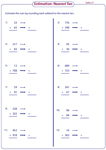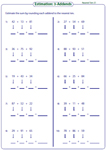Estimate the Sum and Difference Worksheets

Estimate the sums and differences in these worksheets by rounding each number to the nearest ten, hundred, thousand or hundred thousand. Front-end estimation method may also be used to estimate the value in some of the worksheets.

Estimate to the nearest ten

Estimate the value by rounding each number to the nearest ten. Level 1 and Level 2 have worksheets to estimate the sum and difference respectively. Level 3 contains mixed operations.Level 1:

Level 2:

Level 3:

Estimate to the nearest hundred

Round each number to the nearest hundred and estimate the sum and difference in all the worksheets.Level 1:

Level 2:

Level 3:

Estimate to the nearest thousand

Estimate the sum or difference by rounding the numbers to the nearest thousand. Level 3 has multi-digit numbers to perform mixed operations.Level 1:

Level 2:

Level 3:

Estimate the large numbers

Each level has three exclusive worksheets to estimate values by rounding each number to its nearest ten thousand and million.Level 1:

Level 2:

Level 3:

Front end estimation: Mixed Review

Use front-end estimation method to round the given numbers and thereby find the sum and difference in each worksheet. Round the numbers to the nearest hundred in level 1 and to the nearest ten thousand in level 2.Level 1:

Level 2:

Estimating sums: Three addends

Round the three addends to the nearest ten in level 1 and to the nearest thousand in level 2 and estimate their sum.Level 1:

Level 2:

Estimate the value

Perform numerical operations and estimate the value in each problem. Round each number to the nearest ten in level 1 and to the nearest hundred in level 2.Level 1:

Level 2:

The whole batch of worksheets on estimating sums and differences can be downloaded instantly.

Related Worksheets